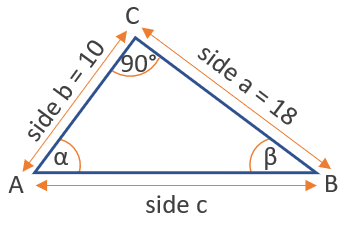# Arctan Calculator

Use this arctan calculator to easily calculate the arctan of a given number. Online arctangent calculation tool to compute the arcus tangens function in degrees or radians. Supports input of decimal numbers (0.5, 6, -1, etc.) and fractions (1/3, 3/4, 1/6, -4/3 etc.).

### Calculation results

26.565051 degrees arctan(0.5) = arctan(0.5) =
Share calculator:

Embed this tool:
get code

## Arctan function

The arctan (a.k.a. arcus tangens) is one of the inverse trigonometric functions (antitrigonometric functions) and is the inverse of the tangent function. It is sometimes written as tan-1(x), but this notation should be avoided as it can cause confusion with an exponent notation. The arctan is used to obtain an angle from the tangent trigonometric ratio, which is the ratio between the side opposite to the angle and the adjacent side of the triangle.

The function spans all real numbers (-∞ - +∞) and so do the results from our calculator. The range of the angle values is usually between -90° and 90°. There are a number of arctan rules, like that tan(arctan(x)) = x, or that arctanα + arctanβ = arctan((α + β) / (1-αβ)), as well as sine of the arctangent: sin(arctan(x)) = x / √(1+x2), which can help you in trigonometry calculations.

## How to calculate the arctan of a number?

The easiest way to calculate it is by using our arctan calculator above, which will output results in both degrees and radians. Other ways require additional information to have been provided such as the values of other trigonometric functions for the same angle or for other angles in a triangle (see example below).

Here is a table of common arctan values:

Common values of the arctan function
-∞ -90° -π/2
-√3 -60° -π/3
-1 -45° -π/4
-1/√3 -30° -π/6
0 0
1/√3 30° π/6
1 45° π/4
√3 60° π/3
+∞ 90° π/2

π is, of course, the mathematical constant about equal to 3.14159.

## Example of using arctan

Given the figure below of a right angle triangle with known side lengths a = 18 and b = 10, and a right angle at point C, how can we find the angle β at point B using the inverse tangent function?Knowing that the tangent of β equals the opposite side divided by the adjacent side, one gets tan(β) = b / a = 10 / 18 = 0.555. Then simply use the inverse function to get β = arctan(0.555) = 29.03° (or 0.507 in radians).

## Calculating arctangent of a fraction

Oftentimes the tangent value will be given or calculated as a simple fraction, e.g. 3/4. While one can use a fraction to decimal converter to convert the fraction into a decimal, our arctangent calculator actually supports direct input of various fractions like 1/2, 1/3, 1/6, 3/4, 4/3, -2/3, and even 0.3/.5 . To compute arctan(3/4) or arctan(4/3) or another x/y fraction, simply enter it into the input field and press "calculate".

For convenience, here is a table of common arctangent values of fraction tangents:

Common arc tan values of fraction tangents